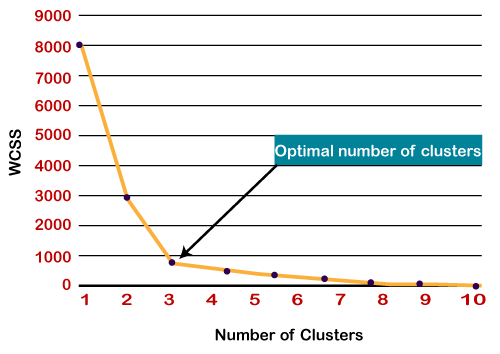# How to find K in K means algorithm?

Machine learning algorithm

Elbow method: Calculate the Within-Cluster-Sum of Squared Errors (WSS) for different values of k, and choose the k for which WSS first starts to diminish. In the plot of WSS-versus-k, this is visible as an elbow.

Silhouette method: The silhouette value measures how similar a point is to its own cluster (cohesion) compared to other clusters (separation).The range of the Silhouette value is between +1 and -1. A high value is desirable and indicates that the point is placed in the correct cluster. If many points have a negative Silhouette value, it may indicate that we have created too many or too few clusters.

The performance of the K means clustering algorithm depends upon the highly efficient cluster that it forms. Since the value of the K is pre-determined, it becomes difficult to choose the number of clusters.

To find the optimal value of k we use the elbow method:-

To find the optimal value of clusters, the elbow method follows the below steps:

1. It executes the K-means clustering on a given dataset for different K values (ranges from 1-10).

2. For every value of K, calculates the WCSS value.

3. Plots a curve between calculated WCSS values and the number of clusters K.

4. The sharp point of bend or a point of the plot looks like an arm, then that point is considered as the best value of K.